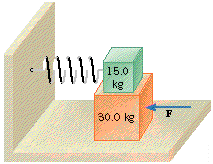# A 30.0 kg block is resting on a flat horizontal table. On topof this block is resting a 15.0 kg block, to which a horizontal spring is attached. The sChardonnay Felix 2021-03-06 Answered
A 30.0 kg block is resting on a flat horizontal table. On topof this block is resting a 15.0 kg block, to which a horizontal spring is attached. The spring constant of the spring is 325 N/m. The coefficient of kinetic friction between the lower block and the table is 0.600, and the coefficient of static friction between the two blocks is 0.900. A horizontal force is applied to the lower block. This force is increasing in such a way as to keep the blocks moving at a constant speed. At the point where the upper block begins to slip on the lower block,determine (a) the amount by which the spring is compressed and (b) the magnitude of the force F.
You can still ask an expert for help

• Questions are typically answered in as fast as 30 minutes

Solve your problem for the price of one coffee

• Math expert for every subject
• Pay only if we can solve itSoosteethicU

At the point where the upper block begins to slip, the spring force is equal to (actually, slightly greater than) the frictional forceJust before this time, the forces acting on each block give rise to an acceleration, which is the same for both blocks
$F-\mu \left(m+M\right)g=Ma$
$\mu mg-kx=ma$
Solving each of these equations for a and equating
$a={\mu }_{s}g-\frac{kx}{m}=\frac{F}{M}-\frac{m+M}{M}{\mu }_{k}g$
Substitute the value for x from above, then solve thisequation for F